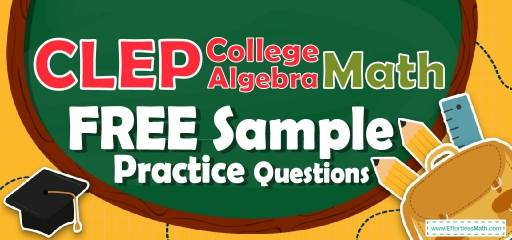# CLEP College Algebra FREE Sample Practice QuestionsPreparing for the CLEP College Algebra test? To do your best on the CLEP College Algebra test, you need to review and practice real CLEP College Algebra questions.  There’s nothing like working on CLEP College Algebra sample questions to hone your math skills and put you more at ease when taking the CLEP College Algebra test. The sample math questions you’ll find here are brief samples designed to give you the insights you need to be as prepared as possible for your CLEP College Algebra test.

Check out our sample CLEP College Algebra practice questions to find out what areas you need to practice more before taking the CLEP College Algebra test!

Start preparing for the 2022 CLEP College Algebra test with our free sample practice questions. Also, make sure to follow some of the related links at the bottom of this post to get a better idea of what kind of mathematics questions you need to practice.

## 10 Sample CLEP College Algebra Practice Questions

1- If $$A={1,2,4,8,16,24}$$ and $$B={1,4,12,24,32,48}$$, how many elements are in $$A∪B$$?

A. 2

B. 3

C. 6

D. 9

E. 12

2- The average of five consecutive numbers is 36. What is the smallest number?

A. 38

B. 36

C. 34

D. 12

E. 8

3- Which of the following numbers is NOT a solution to the inequality $$2x-5≥3x-1$$?

A. $$-2$$

B. $$-4$$

C. $$-5$$

D. $$-8$$

E. $$-10$$

4- If the following equations are true, what is the value of $$x$$?
$$a=\sqrt{3}$$
$$4a=\sqrt{4x}$$

A. 2

B. 3

C. 6

D. 12

E. 14

5- If $$\sqrt{4m-3}=m$$, what is (are) the value(s) of m?

A. 0

B. 1

C. 1, 3

D. $$-1, 3$$

E. $$-1, -3$$

6- When a number is subtracted from 28 and the difference is divided by that number, the result is 3. What is the value of the number?

A. 2

B. 4

C. 7

D. 12

E. 24

7- An angle is equal to the one-ninth of its supplement. What is the measure of that angle?

A. 9

B. 18

C. 25

D. 60

E. 90

8- If tan $$\theta=\frac{5}{12}$$ and sin $$\theta> 0$$, then cos $$\theta=$$?

A. $$-\frac{5}{13}$$

B. $$\frac{12}{13}$$

C. $$\frac{13}{12}$$

D. $$-\frac{12}{13}$$

E. 0

9- Which of the following has the same period and two times the amplitude of the graph
$$y =$$cos $$x$$?

A. $$y=$$cos $$2x$$

B. $$y=$$cos $$(x+2)$$

C. $$y=4$$ cos⁡ $$2x$$

D. $$y=2+2$$ cos $$x$$

E. $$y=4+$$cos $$x$$

10- If $$y=nx+2$$, where n is a constant, and when $$x=6, y=14$$, what is the value of y when $$x=10$$?

A. 10

B. 12

C. 18

D. 22

E. 24

## Best CLEP College Algebra Prep Resource for 2022

1- D
The union of $$A$$ and $$B$$ is: $$A∪B={1,2,4,8,12,16,24,32,48}$$. There are 9 elements in $$A∪B$$.

2- C
Let $$x$$ be the smallest number. Then, these are the numbers: $$x, x+1, x+2, x+3, x+4$$
average$$=\frac{sum \ of \ terms}{number \ of \ terms}⇒36=\frac{x+(x+1)+(x+2)+(x+3)+(x+4)}{5}⇒36=\frac{5x+10}{5}$$
$$⇒180=5x+10 ⇒170= 5x⇒x=34$$

3- A
Subtracting $$2x$$ and adding 5 to both sides of $$2x – 5 ≥ 3x – 1$$ gives $$-4 ≥ x$$. Therefore, $$x$$ is a solution to $$2x – 5 ≥ 3x – 1$$ if and only if $$x$$ is less than or equal to $$-4$$ and x is NOT a solution to$$2x – 5 ≥ 3x – 1$$ if and only if $$x$$ is greater than $$-4$$. Of the choices given, only $$-2$$ is greater than \9-4\) and, therefore, cannot be a value of $$x$$.

4- D
Given the two equations, substitute the numerical value of a into the second equation to solve for $$x$$. $$a=\sqrt{3}, 4a=\sqrt{4x}$$
Substituting the numerical value for an into the equation with x is as follows.
$$4(\sqrt{3})=\sqrt{4x}$$,From here, distribute the 4. $$4\sqrt{3}=\sqrt{4x}$$
Now square both sides of the equation. $$(4\sqrt{3})^2=(\sqrt{4x})^2$$
Remember to square both terms within the parentheses. Also, recall that squaring a square root sign cancels them out. $$4^2 \sqrt{3}^2=4x, 16(3)=4x, 48=4x, x=12$$

5- C
First square both sides of the equation to get $$4m-3=m^2$$
Subtracting both sides by $$4m-3$$ gives us the equation $$m^2-4m+3=0$$
Here you can solve the quadratic equation by factoring to get $$(m-1)(m-3)=0$$
For the phrase $$(m-1)(m-3)$$ to equal zero, $$m=1$$ or $$m=3$$

6- C
Let $$x$$ be the number. Write the equation and solve for $$x. (28-x)÷x= 3$$
Multiply both sides by $$x$$. $$(28-x)= 3x$$, then add x both sides. $$28=4x$$, now divide both sides by 4.$$x=7$$

7- B
The sum of supplement angles is 180. Let $$x$$ be that angle. Therefore, $$x+9x=180$$
$$10x=180$$, divide both sides by 10: $$x=18$$

8- B
tan$$θ=\frac{opposite}{adjacent}$$
tan$$θ=\frac{5}{12}⇒$$ we have the following right triangle. Then
$$c=\sqrt{5^2+12^2 }=\sqrt{25+144}=\sqrt{169}=13$$
cos$$θ=\frac{adjacent}{hypotenuse}=\frac{12}{13}$$
$$\img{https://appmanager.effortlessmath.com/public/images/questions/ppp.png }$$

9- D
The amplitude in the graph of the equation $$y=$$acosb$$x$$ is a. (a and b are constant)
In the equation $$y=$$cos$$x$$, the amplitude is 2 and the period of the graph is $$2π$$.
The only choice that has two times the amplitude of graph $$y =$$ cos $$x$$ is $$y=2+2$$ cos $$x$$
They both have an amplitude of 2 and a period of $$2π$$.

10- D
Substituting 6 for $$x$$ and 14 for $$y$$ in $$y = nx+2$$ gives $$14=(n)(6)+2$$,
which gives $$n=2$$. Hence, $$y=2x+2$$. Therefore, when $$x = 10$$, the value of $$y$$ is
$$y=(2)(10)+2 = 22$$.

Looking for the best resource to help you succeed on the CLEP College Algebra test?

## The Best Books to Ace the CLEP College Algebra Test

### What people say about "CLEP College Algebra FREE Sample Practice Questions - Effortless Math: We Help Students Learn to LOVE Mathematics"?

No one replied yet.

X
23% OFF

Limited time only!

Save Over 23%

SAVE $5 It was$21.99 now it is \$16.99